Let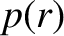be the equilibrium plasma pressure profile. The equilibrium force balance relation (see Section 2.25)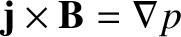(14.30)

gives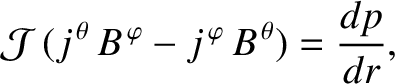(14.31)

where use has been made of Equations (14.5) and (14.7). The previous equation reduces to the Grad-Shafranov equation [19,26,32],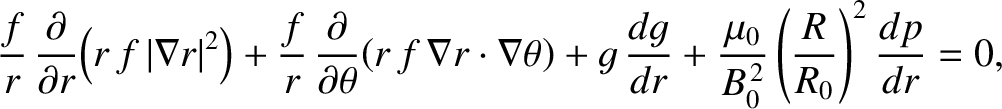(14.32)

where use has been made of Equations (14.20), (14.21), (14.28), and (14.29). The Grad-Shafranov equation can be written in the alternative form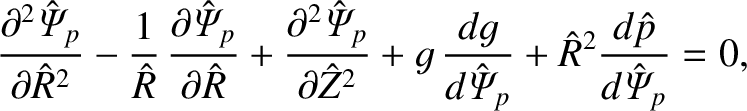(14.33)

where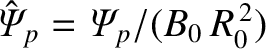,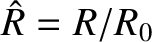,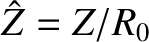, and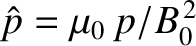.